×#### Thank you for registering.

One of our academic counsellors will contact you within 1 working day.

Click to Chat

1800-1023-196

+91-120-4616500

CART 0

• 0

MY CART (5)

Use Coupon: CART20 and get 20% off on all online Study Material

ITEM
DETAILS
MRP
DISCOUNT
FINAL PRICE
Total Price: Rs.

There are no items in this cart.
Continue Shopping• Complete JEE Main/Advanced Course and Test Series
• OFFERED PRICE: Rs. 15,900
• View Details

```Chapter 8: Quadratic Equations Exercise – 8.8

Question: 1

The speed of a boat in still water is 8km/hr. It can go 15 km upstream and 22 km downstream in 5 hours. Find the speed of the stream.

Solution:

Let the speed of stream be x km/hr,

Then, speed downstream = (8 + x) km/hr

Therefore, Speed upstream = (8 - x) km/hr

Time taken by the boat to go 15 km upstream 15/(8 - x)hr

Time taken by the boat to return 22 km downstream = 22/(8 + x)hr Acc.

To the question, the boat returns to the same point in 5 hr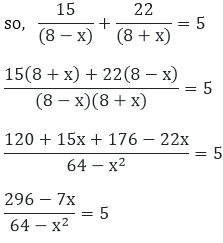5x2 - 7x + 296 - 320 = 0

5x2 - 7x - 24 = 0

5x2 - 15x + 8x - 24 = 0

5x(x - 3) + 8(x - 3) = 0

(x - 3)(5x + 8) = 0

x = 3, x = - 8/5

Since the speed of the stream can never be negative, hence the speed of the stream is 3 km/hr.

Question: 2

A train traveling at a uniform speed for 360 km, would have taken 48 minutes less to travel the same distance if its speed were 5 km/hr more. Find the original speed of the train.

Solution:

Let the usual speed of train be x km/hr

Then, increased speed of the train = (x + 10) km/hr

Time taken by the train under usual speed to cover 360 km = 360/x hr.

Time taken by the train under increased speed to cover 360 km = 360/(x + 5) hr.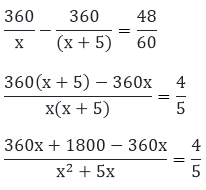1800(5) = 4(x2 + 5x)

9000 = 4x2 + 20x

4x2 + 20x - 9000 = 0

x2 + 5x - 2250 = 0

x2 + 50x - 45x - 2250 = 0

x(x + 50) - 45(x + 50) = 0

(x + 50)(x - 45) = 0

x = - 50 or x = 45

Since, the speed of the train can never be negative. Hence, original speed of train is 45 km/hr.

Question: 3

A fast train takes one hour less than a slow train for a journey of 200 km. If the speed of the slow train is 10 km/hr less than that of the fast train, find the speed of the two train.

Solution:

Let the speed of the fast train be x km/hr

Then, the speed of the slow train be = (x -10) km/hr

Time taken by the fast train to cover 200 km = 200/x hr

Time taken by the slow train to cover 200 km = 200/(x - 10) hr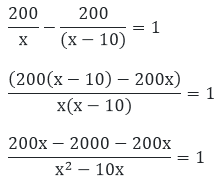x2- 10x = - 2000

x2 - 10x + 2000 = 0

x2 - 50x + 40x + 2000 = 0

x(x - 50) + 40(x - 50) = 0

(x - 50)(x + 40) = 0

x = 50 or x = - 40

Since, the speed of train can never be negative.

Therefore, x = 50

So, speed of the fast train is 50 km/hr

And speed of slow train (50 - 10) = 40 km/hr (given speed of slow train is 10km/hr less than fast train)

Question: 4

A passenger train takes one hour less for a journey of 150 km if its speed is increased 5 km/hr from its usual speed. Find the usual speed of the train.

Solution:

Let the usual speed of train be x km/hr

Then, increased speed of the train = (x + 5) km/hr

Time taken by the train under usual speed to cover 150 km = 150/x hr

Time taken by the train under increased speed to cover 150 km = 150(x + 5)hr750 = x2 + 5x

x2 + 5x - 750 = 0

x2 - 25x + 30x -750 = 0

x(x - 25) + 30(x - 25) = 0

(x - 25) (x + 30) = 0

x = 25  or x = -30 Since, the speed of the train can never be negative

Therefore, the usual speed of the train is x = 25 km/hr

Question: 5

The time taken by a person to cover 150 km was 2.5 hrs more than the time taken on the return journey. If he returned at the speed of 10 km/hr more than the speed of going, what was the speed per hour in each direction?

Solution:

Let the ongoing speed of person be x km/hr,

Then the returning speed of the person is = (x + 10) km/hr

Time taken by the person in going direction to cover 150 km = 150/x hr

Time taken by the person in returning direction to cover 150 km = 150/(x + 10)hr

Therefore,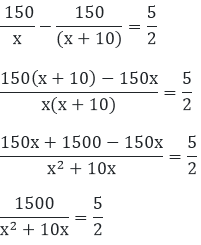3000 = 5x2 + 50x

5x2 + 50x - 3000 = 0

5(x2 + 10x - 600) = 0

x2 + 10x - 600 = 0

x2 - 20x + 30x - 600 = 0

x(x - 20) + 30(x - 20) = 0

(x - 20)(x + 30) = 0

x = 20  or x = -30 Since the speed of train can never be negative

Therefore, x = 20 Then, (x + 10) (20 + 10) = 30

Hence, ongoing speed of person is 20km/hr.

And returning speed of the person is 30 km/hr.

Question: 6

A plane left  40 minutes late due to bad weather and in order to reach the destination, 1600 km away in time, it had to increase its speed by 400 km/hr from its usual speed.. Find the usual speed of the plane.

Solution:

Let the usual speed of plane be x km/hr,

Then the increased speed of the plane is = (x + 4000) km/hr

Time taken by the plane under usual speed to cover 1600 km = 1600/hr

Time taken by the plane under increased speed to cover 1600 km = 1600/(x + 400)hr

Therefore,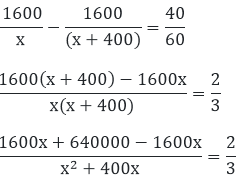1920000 = 2x2 + 800x

2x2 + 800x - 1920000 = 0

2(x2+ 400x - 960000) = 0

x2 + 400x - 960000 = 0

x2 - 800x + 1200x - 960000 = 0

x(x - 800) + 1200(x - 800) = 0

(x - 800)(x + 1200) = 0

x = 800 or x = -1200

Since the speed of the train can never be negative.

Therefore, the usual speed of the train is 800 km/hr.

Question: 7

An aero plane takes 1 hour less for a journey of 1200 km if its speed is increased by 100 km/hr from its usual speed of the plane. Find its usual speed.

Solution:

Let the usual speed of plane be x km/hr,

Then the increased speed of the plane is = (x + 100) km/hr

Time taken by the plane under usual speed to cover 1200 km = 1200/x hr

Time taken by the plane under increased speed to cover 1200 km = 1200/(x + 100)hr

Therefore,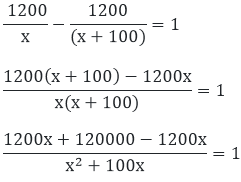120000 = x2 + 100x

x2 + 100x - 120000 = 0

x2 - 300x + 400x - 120000 = 0

x(x - 300) + 400(x - 300) = 0

x = 300 or  x = - 400 Since, the speed of the aero plane can never be negative

Hence, the usual speed of train is 300 km/hr

Question: 8

A train covers a distance of 90 km at a uniform speed. Had the speed been 15 km/hr more, it would have taken 30 minutes less for the journey. Find the original speed of the train.

Solution:

Let the usual speed of train be x km/hr,

Then the increased speed of the train is = (x + 15) km/hr

Time taken by the train under usual speed to cover 90km = 90/x hr

Time taken by the train under increased speed to cover 90 km = 90/(x + 15)hr

Therefore,2700 = x2 + 15x

x2 + 15x - 2700 = 0

x2 - 45x + 60x - 2700 = 0

x(x - 45) + 60(x - 45) = 0

(x - 45)(x + 60) = 0

x = 45 or x = - 60

Since, the speed of the train can never be negative

Therefore, the original speed of the train is 45 km/hr.

Question: 9

A train travels 360 km at a uniform speed. If the speed had been 5 km/hr more, it would have taken 1 hr less for the same journey. Find the speed of the train.

Solution:

Let the usual speed of train be x km/hr,

Then the increased speed of the train is = (x + 5) km/hr

Time taken by the train under usual speed to cover 360 km = 360/x hr

Time taken by the train under increased speed to cover 360 km = 360/(x + 5)hr

Therefore,1800 = x2 + 5x

x2 + 5x - 1800 = 0

x2 - 40x + 45x - 1800 = 0

x(x - 40) + 45(x - 40) = 0

(x + 45)(x - 40) = 0

x = 40 or x = - 45

Since the speed of the train can never be negative

Hence, the original speed of the train is 40 km/hr.

Question: 10

An express train takes 1 hour less than a passenger train to travel 132 km between my sore and Bangalore. If the average speed of the express train is 11 km/hr more than that of the passenger train, find the average speed of the two trains.

Solution:

Let the usual speed of train be x km/hr,

Then the increased speed of the train is = (x + 11) km/hr

Time taken by the passenger train to cover 132 km = 132/x hr

Time taken by the express train to cover 132 km = 132/(x + 11)hr

Therefore,1452 = x2 + 11x

x2 + 11x - 1452 = 0

x2 - 33x + 44x - 1452 = 0

x(x - 33) + 44(x - 33) = 0

(x - 33)(x + 44) = 0

x = 33 or  x = - 44 Since, speed of train can never be negative

Therefore, speed of passenger train = 33 km/hr.

And speed of express train, = (x + 11) = (33 + 11) = 44 km/hr.

Question: 11

An aero plane left 50 minutes later than its scheduled time, and in order to reach the destination, 1250 km away, in time, it had to increase its speed by 250 km/hr from its usual speed. Find its usual speed.

Solution:

Let the usual speed of aero plane be x km/hr,

Then the increased speed of the aero plane is = (x + 250) km/hr

Time taken by the aero plane under usual speed to cover 1250 km = 1250/x hr

Time taken by the aero plane under increased speed to cover 1250 km = 1250/(x + 250)hr

Therefore,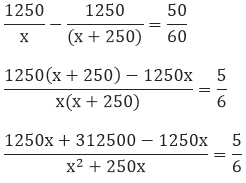1875000 = 5x2 + 1250x

5x2 + 1250x - 1875000 = 0

5(x2 + 1250x - 375000) = 0

X2 + 250x - 375000 = 0

X2 - 500x + 750x - 375000 = 0

X(x - 500) + 750(x - 500) = 0

(x - 500)(x + 750) = 0

X = 500 or x = - 750

Since, the speed of the aero plane can never be negative,

Therefore, the usual speed of the train = 500 km/hr.

Question: 12

While boarding an aero plane, a passenger got hurt. The pilot showing promptness and concern, made arrangements to hospitalize the injured and so the plane started late by 30 minutes to reach the destination, 1500 km away in time, the pilot increased the speed by 100 km/hr. Find the original speed /hour of the plane.

Solution:

Let the usual speed of aero plane be x km/hr,

Then the increased speed of the aero plane is = (x + 100) km/hr

Distance to be travelled = 1500 km.

Time taken to reach the destination at original speed, t1 = 1500/x hr

Time taken to reach the destination at increasing speed, t2 = 1500/(x + 100)hr Acc.

To the question, t1 - t2 = 30 min.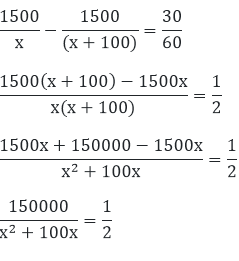300000 = x2 + 100x

x2 + 100x - 300000 = 0

x2 + 600x - 500x - 300000 = 0

x(x + 600) - 500(x + 600) = 0

(x - 500)(x + 600) = 0

x = 500  or x = - 600 Since, the speed of plane can never be negative

Therefore, the original speed of the plane is 500 km/hr.

Question: 13

A motor boat whose speed in still water 18 km/hr takes 1 hour more to go 24 km up stream than to return downstream to the same point. Find the speed of the stream.

Solution:

Let the usual speed of stream be x km/hr,

Speed of the boat in still water is = 18 km/hr

Distance to be travelled = 24 km.

Speed of the boat upstream = speed of the boat in still water – speed of the stream = (18 - x) km/hr

Speed of the boat downstream = speed of the boat in still water + speed of the stream = (18 + x) km/hr. Time of upstream journey, t1 = 24/(18 - x) km/hr

Time of downstream journey, t2 = 24/(18 + x) km/hr Acc.

To the question, t1 – t2 = 1hr.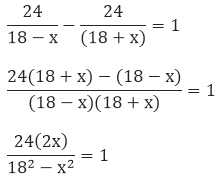48x = 324 - x2

x2 + 48x - 324 = 0

x2 + 54x - 6x - 324 = 0

x(x + 54) - 6(x + 54) = 0

(x - 6)(x +54) = 0

x = 6  or x = – 54

Since the speed can never be negative. Therefore the speed of stream is 6 km/hr.
```### Course Features

• 728 Video Lectures
• Revision Notes
• Previous Year Papers
• Mind Map
• Study Planner
• NCERT Solutions
• Discussion Forum
• Test paper with Video Solution